Ex 13.6

Chapter 13 Class 9 Surface Areas and Volumes (Term 2)
Serial order wise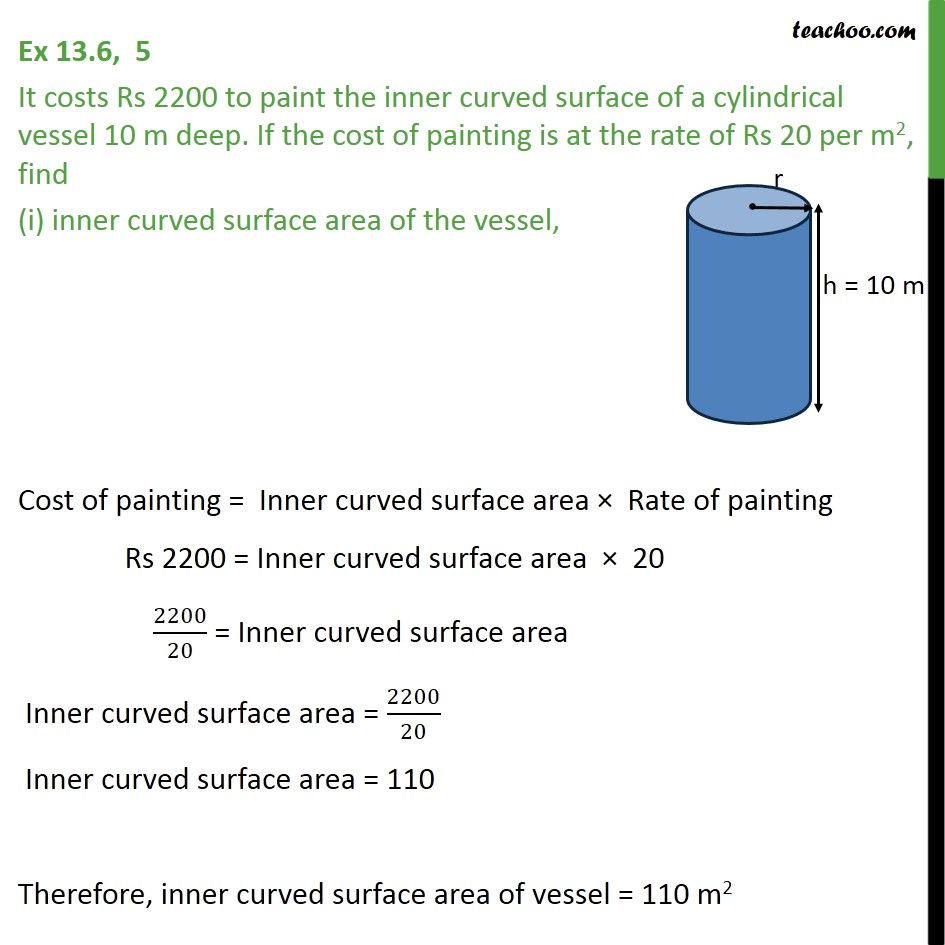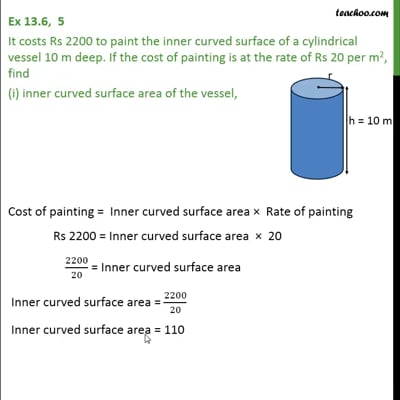This video is only available for Teachoo black users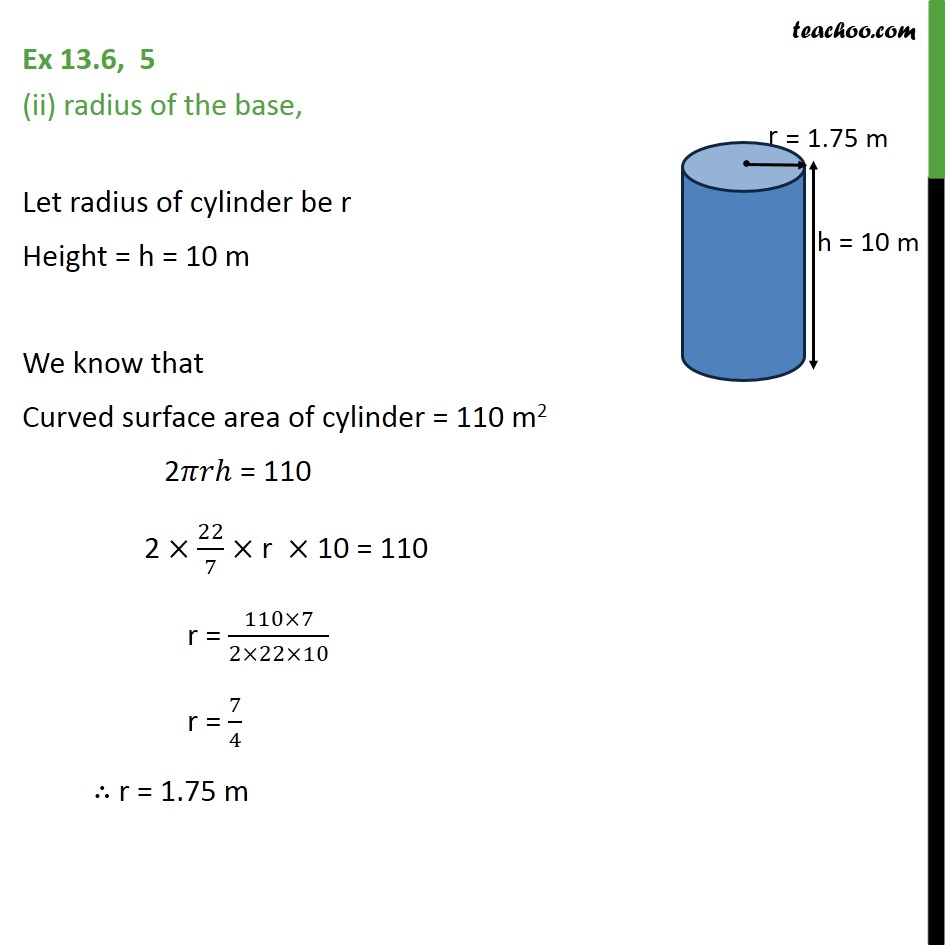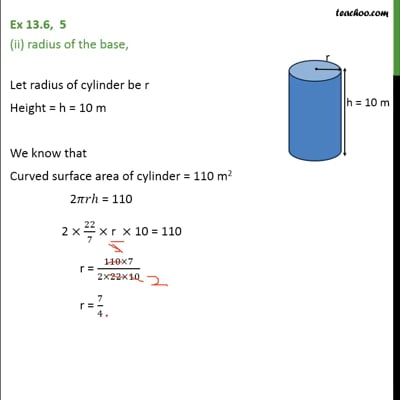This video is only available for Teachoo black users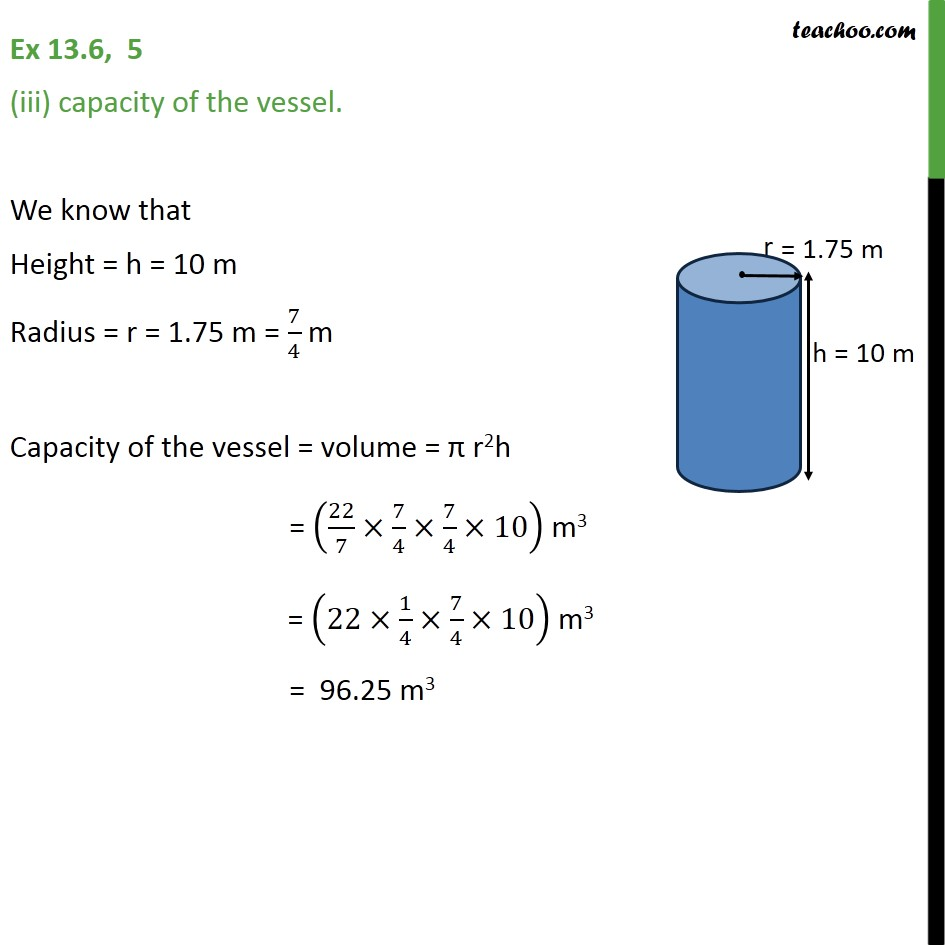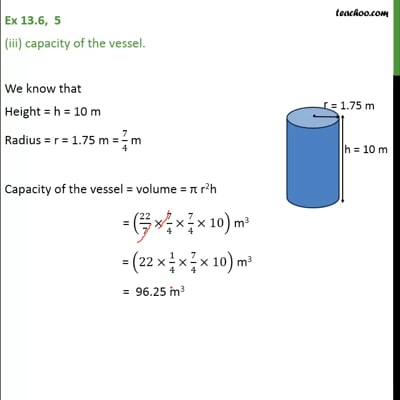This video is only available for Teachoo black users

### Transcript

Ex 13.6, 5 It costs Rs 2200 to paint the inner curved surface of a cylindrical vessel 10 m deep. If the cost of painting is at the rate of Rs 20 per m2, find (i) inner curved surface area of the vessel, Cost of painting = Inner curved surface area Rate of painting Rs 2200 = Inner curved surface area 20 2200/20 = Inner curved surface area Inner curved surface area = 2200/20 Inner curved surface area = 110 Therefore, inner curved surface area of vessel = 110 m2 Ex 13.6, 5 (ii) radius of the base, Let radius of cylinder be r Height = h = 10 m We know that Curved surface area of cylinder = 110 m2 2 = 110 2 22/7 r 10 = 110 r = (110 7)/(2 22 10) r = 7/4 r = 1.75 m Ex 13.6, 5 (iii) capacity of the vessel. We know that Height = h = 10 m Radius = r = 1.75 m = 7/4 m Capacity of the vessel = volume = r2h = (22/7 7/4 7/4 10) m3 = (22 1/4 7/4 10) m3 = 96.25 m3